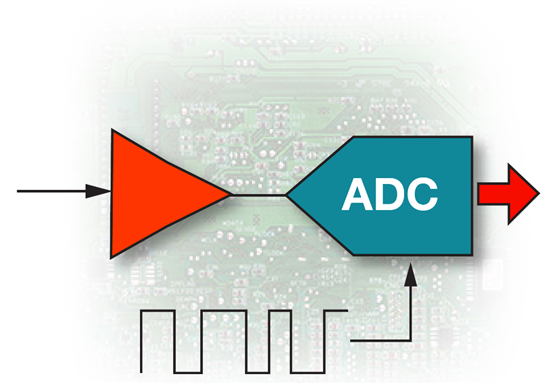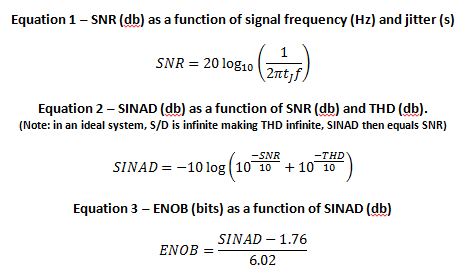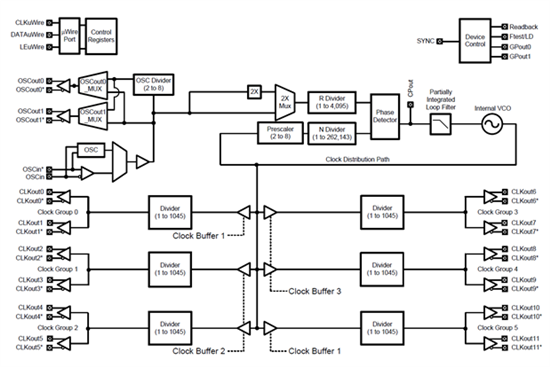# Clocking sampled systems to minimize jitter

As many of you know, jitter (which is clock edge uncertainty) is a bad thing that leads to increased noise and degradation of a data converter’s ENOB.For example, if we have a system that requires an ENOB of 14 (minimum) bits at 100 MHz, we would require a clock with less than 80 femtoseconds of jitter! This can be calculated assuming an ideal system with no distortion, which makes the SINAD and SNR numbers equivalent (see eq. 2).

Next, using an ENOB of 14, we can calculate the minimum SNR at roughly 86 db. Using that in equation 1 yields the roughly 80 fs number for tJ.In digital signal processing, there needs to be a correlation between the sample clock and the processing clock.  That is, the samples, whether decimated or used at full rate, must be processed at a multiple of that rate and be phase coherent.  This requires a “master” clock from which all other clocks in the system are derived.

You can accomplish this using a temperature compensated crystal oscillator (TCXO) and a low phase noise PLL to multiply the master clock to a much higher frequency.  Then you can divide down this new high-frequency clock to provide the remaining system clocks, which all are related to the master.  In this fashion, the sample clocks, as well as the various digital processing clocks, are all correlated to one another.

There are many clocking solutions available today, but many require clock buffers or other methods of distributing the clocks, which can degrade overall jitter performance.  You can use a device like the LMK03806, which combines a master clock generator and clock distribution (with drivers) all in the same device (see figure 1 below), to overcome this. The device has less than 50 fs RMS jitter (1.875 MHz to 20 MHz) while running at 300 MHz. You can also program the outputs to support LVDS, LVPECL or LVCMOS and synchronize them to have a common rising edge.Figure 1 – Block diagram of LMK03806 with clock generator, clock dividers and drivers.

So the next time you’re designing a sampled system, remember to consider the jitter of your clocks as this will impact the overall dynamic range.

If you liked this, be sure to check out more of my blogs on topics ranging from how to increase the dynamic performance of your radar system to  a discussion on the challenges of high-speed communications.